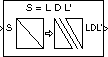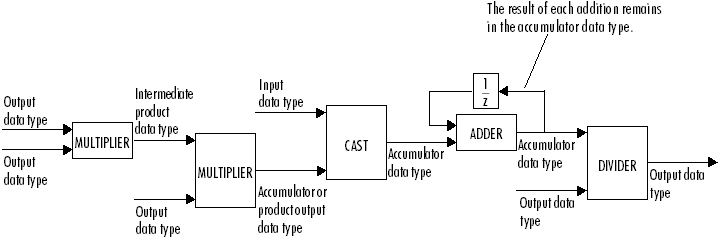Documentation

# LDL Factorization

Factor square Hermitian positive definite matrices into lower, upper, and diagonal components

## Library

Math Functions / Matrices and Linear Algebra / Matrix Factorizations

`dspfactors`## Description

The LDL Factorization block uniquely factors the square Hermitian positive definite input matrix S as

`$S=LD{L}^{*}$`

where L is a lower triangular square matrix with unity diagonal elements, D is a diagonal matrix, and L* is the Hermitian (complex conjugate) transpose of L. Only the diagonal and lower triangle of the input matrix are used, and any imaginary component of the diagonal entries is disregarded.

The block's output is a composite matrix with lower triangle elements lij from L, diagonal elements dij from D, and upper triangle elements uij from L*. The output format is shown below for a 5-by-5 matrix.LDL factorization requires half the computation of Gaussian elimination (LU decomposition), and is always stable. It is more efficient than Cholesky factorization because it avoids computing the square roots of the diagonal elements.

The algorithm requires that the input be square and Hermitian positive definite. When the input is not positive definite, the block reacts with the behavior specified by the Non-positive definite input parameter.

### Fixed-Point Data Types

The following diagram shows the data types used within the LDL Factorization block for fixed-point signals.You can set the intermediate product, product output, accumulator, and output data types in the block dialog as discussed below.

The output of the second multiplier is in the product output data type when the input is real. When the input is complex, the result of the multiplication is in the accumulator data type. For details on the complex multiplication performed, see Multiplication Data Types.

## Examples

LDL decomposition of a 3-by-3 Hermitian positive definite matrix:## Parameters

Main Tab

Non-positive definite input

Specify the action when nonpositive definite matrix inputs occur:

• `Ignore` — Proceed with the computation and do not issue an alert. The output is not a valid factorization. A partial factorization is present in the upper left corner of the output.

• `Warning` — Display a warning message in the MATLAB® Command Window, and continue the simulation. The output is not a valid factorization. A partial factorization is present in the upper left corner of the output.

• `Error` — Display an error dialog and terminate the simulation.

Data Types Tab

Rounding mode

Specify the rounding mode for fixed-point operations as one of the following:

• `Floor`

• `Ceiling`

• `Convergent`

• `Nearest`

• `Round`

• `Simplest`

• `Zero`

For more details, see rounding mode.

Saturate on integer overflow

When you select this parameter, the block saturates the result of its fixed-point operation. When you clear this parameter, the block wraps the result of its fixed-point operation. For details on `saturate` and `wrap`, see overflow mode for fixed-point operations.

Intermediate product

Specify the intermediate product data type. As shown in Fixed-Point Data Types, the output of the multiplier is cast to the intermediate product data type before the next element of the input is multiplied into it. You can set it to:

• A rule that inherits a data type, for example, `Inherit: Same as input`

• An expression that evaluates to a valid data type, for example, `fixdt(1,16,0)`

Click the buttonto display the Data Type Assistant, which helps you set the Product output parameter.

Product output

Specify the product output data type. See Fixed-Point Data Types and Multiplication Data Types for illustrations depicting the use of the product output data type in this block. You can set it to:

• A rule that inherits a data type, for example, `Inherit: Inherit via internal rule`. For more information on this rule, see Inherit via Internal Rule.

• A rule that inherits a data type, for example, `Inherit: Same as input`.

• An expression that evaluates to a valid data type, for example, `fixdt(1,16,0)`

Click the buttonto display the Data Type Assistant, which helps you set the Product output parameter.

Accumulator

Specify the accumulator data type. See Fixed-Point Data Types for illustrations depicting the use of the accumulator data type in this block. You can set this parameter to:

• A rule that inherits a data type, for example, `Inherit: Inherit via internal rule`. For more information on this rule, see Inherit via Internal Rule.

• A rule that inherits a data type, for example, `Inherit: Same as input`.

• A rule that inherits a data type, for example, ```Inherit: Same as product output```.

• An expression that evaluates to a valid data type, for example, `fixdt(1,16,0)`

Click the buttonto display the Data Type Assistant, which helps you set the Accumulator parameter.

Output

Specify the output data type. See Fixed-Point Data Types for illustrations depicting the use of the output data type in this block. You can set it to:

• A rule that inherits a data type, for example, `Inherit: Same as input`

• An expression that evaluates to a valid data type, for example, `fixdt(1,16,0)`

Click the buttonto display the Data Type Assistant, which helps you set the Output parameter.

Lock data type settings against changes by the fixed-point tools

Select this parameter to prevent the fixed-point tools from overriding the data types you specify on the block mask.

## References

Golub, G. H., and C. F. Van Loan. Matrix Computations. 3rd ed. Baltimore, MD: Johns Hopkins University Press, 1996.

## Supported Data Types

PortSupported Data Types

S

• Double-precision floating point

• Single-precision floating point

• Fixed point (signed only)

• 8-, 16-, and 32-bit signed integers

LDL`'`

• Double-precision floating point

• Single-precision floating point

• Fixed point (signed only)

• 8-, 16-, and 32-bit signed integers

 Cholesky Factorization DSP System Toolbox LDL Inverse DSP System Toolbox LDL Solver DSP System Toolbox LU Factorization DSP System Toolbox QR Factorization DSP System Toolbox

See Matrix Factorizations for related information.

Watch now